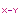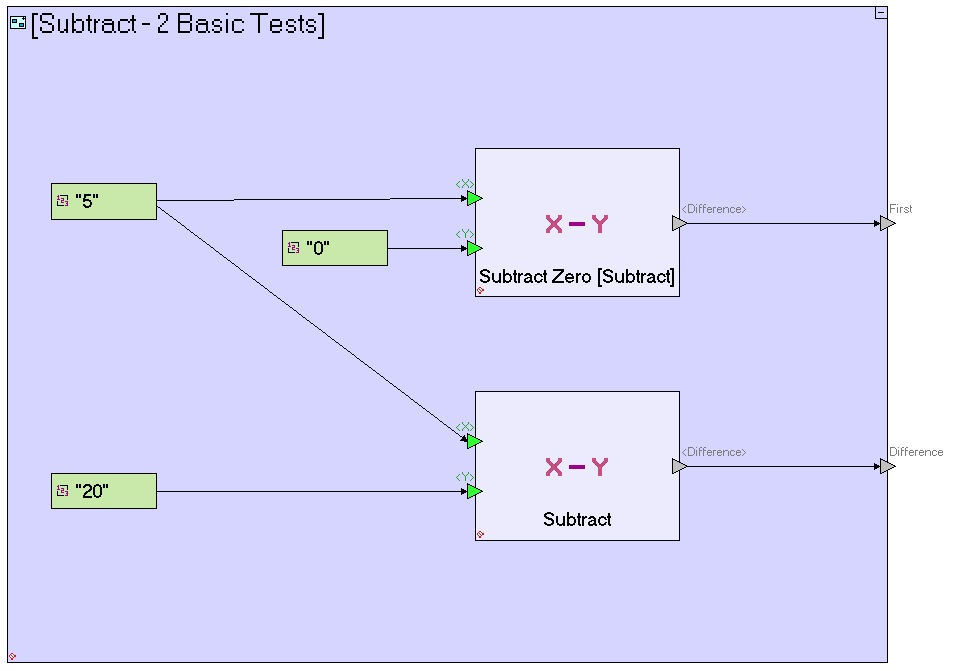Subtract - Subtract two numbers

This action calculates the difference between two numbers.

Triggers

<X> (non-repetitive numeric triggers [required]): The subtractee (the number from which <Y> is subtracted).

<Y> (non-repetitive numeric triggers [required]): The subtractor (the number subtracted from <X>).

Exit

<Difference> (non-repetitive numeric exit [required]): The calculated difference <X> - <Y>.

Usage Examples

“Common/Templates/Math/Subtract/Test1/Subtract - 2 Basic Tests”: